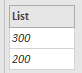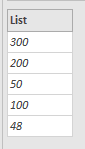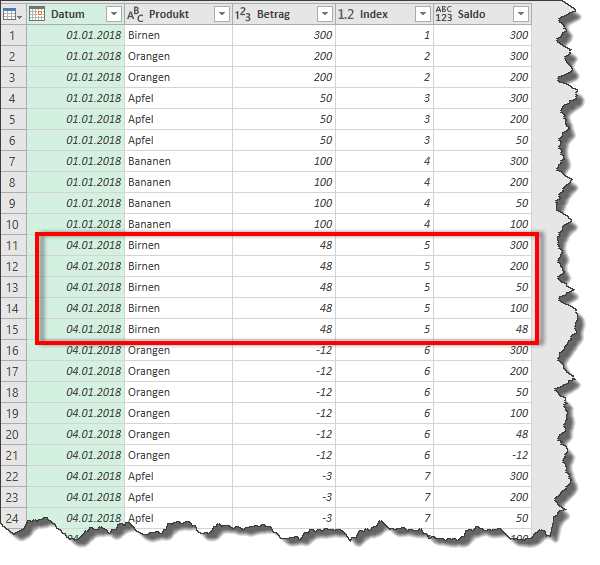# Grouped running total in Power Query

Hits: 11443

The following article was written on the occasion of a Power BI User Meeting. It certainly makes more sense to solve the problem using DAX. But still the question has not let go of me and I show you how a grouped running total can be created in Power Query.

# Introduction

For today’s example I have created a small table which serves as a starting point.

The table includes stock movements from 1.1. to 22.1.2018 for the four fruit varieties apples, bananas, pears and oranges. The aim is to create a grouped balance for each fruit variety.

With DAX, this task is very easy to solve thanks to the time intelligence. This task is also possible in Power Query, but requires a few extra steps.

## 1. Form a running total

I loaded the table into Power Query and renamed the query to “Stock Movements”. The next step was to define the data types. I always do this step manually to make sure that the data types are set correctly.

The next step is to create a new query referring to the first query “stock movements”. I sorted the dates by date.

In order to form the continuous balance, we need an index column, starting at 1, which you can easily create via the interface.

This is followed by the heart of the code, the formation of the running total. Enter the following code into a new, user-defined column:

The new column contains two functions.

### List.Range

The inner, i.e. the second, function, List.range, forms an appended list from the column[Amount]. It looks like this for the first line:The second line then contains the first and the entry of the current line:And on the fifth line:As soon as I expand the list, my query changes into a table that repeats the entries.### List.Sum

As a final step, List.sum comes into play. This function forms the running total from the column just created.

Since List.sum needs a list as input, I concatenated the two functions and thus also avoided the extension of the columns.

### Result:

The result is as follows:

Now the index column must be deleted and the data type for the column[Balance] must be adjusted, and the table with the continuous balance is finished.

Here’s the code.

## 2. Grouped running total

The solution shown for the running total will be used later for the grouped running total. Unfortunately, I cannot create a grouped running total directly from the [Total] column using the grouping function. For this we need the support of “M”, the code language behind Power Query.

The first step is to create a new query referring to the basic query “stock movements”.

The table is then grouped by product. As aggregation I have selected “All rows” and named the new column as “Data”. This step can be conveniently created via the interface.

The M code behind it looks like this:

`#"Grouped Rows" = Table.Group(Source, {"Product"}, {{"Data", each _, type table}})`

That is how we formed the head of the command. Now we have to get Power Query to group the new column [Data] as desired and form the grouped running total.
To do this, we need to adjust the last part of the command, “each _”, and provide a new created table as input. To do this, we use the code from the first example, easily adapt it and convert it into a function.

### Create function

The first step is to change the data table to an input variable.

This is done with `(Input as table) =>`

The next three steps remain unchanged.

``` Sorting =         Table.Sort(Quelle,{{"Date", Order.Ascending}}), added_Index =      Table.AddIndexColumn(Sorting, "Index", 1, 1), cumulativ_total =  Table.AddColumn(added_Index, "Total", each List.Sum(List.Range(added_Index[Amount],0,[Index]))), ch_Type =         Table.TransformColumnTypes(cumulativ_total,{{"Total", Int64.Type}}), removed_column =  Table.RemoveColumns(ch_Type,{"Index"}) in removed_column```

We only want to get back one column as the result for the grouping. Therefore, we create a list from the[Total] column.

`extract_total = cumulativ_total[Total]`

A list cannot be formatted, so there is no need to specify the data type or the last step, since we only have one column or list.

The entire code of the “fn_cumulative_Total” function looks like this.

### Use new function in grouping

Now we change the last part of the created grouping function.

Instead of “each _” we write:

```// Function Call (Input as table) as table => // data for function let Call_Function = fn_cumulative_Total(Input), // End of Function Call ```

The result returned, the continuous balance, must still be converted into a column and merged with the other columns.

```result = Table.FromColumns( Table.ToColumns(Input){Call_Function}, Value.Type(Table.AddColumn( Input, "Total", each null, type number))) in result ```

Now we have included a grouped table containing the result in the [Data] column:

After extending the[Data] column, we see that the function continuously sums up all the movements of a product. As soon as a new product starts, a new total is added.

and here is the complete code for forming the grouped running total.

# Conclusion

Existing functions such as grouping can be extended and adapted with the aid of “M” code. I hope the article will help you with such and similar use cases.

More articles about Power Query can be found in the Power Query Online Buch.

Happy Querying!

Dear readers, I write my articles for you. It is my ambition to provide you with qualitatively appealing content. Please take a minute and give me a review or comment below. Thank you very much.

[yasr_visitor_multiset setid=2]

### 4 thoughts on “Grouped running total in Power Query”

•12. November 2018 at 21:00

Any chance you can share the PBIX file?

•30. January 2019 at 11:33

•9. January 2019 at 20:18
•